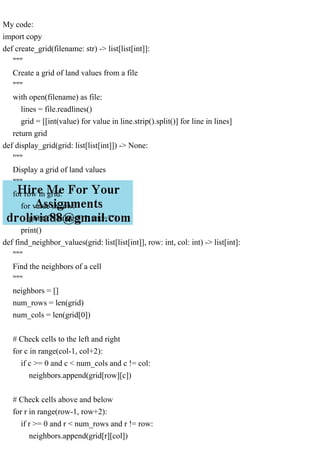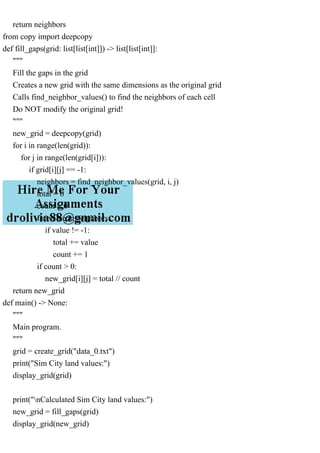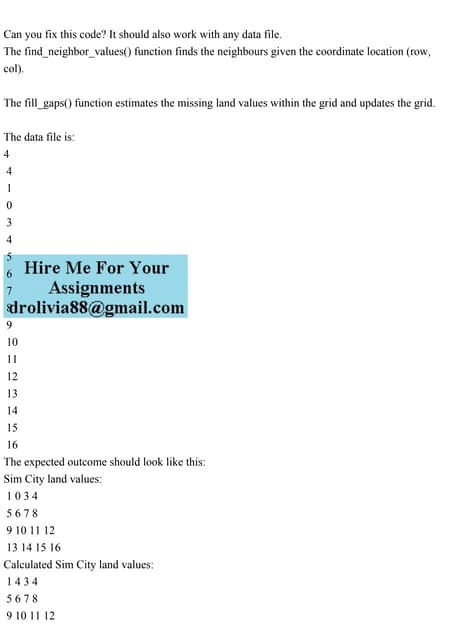AnúncioAnúncioPróximos SlideSharesCan you fix this code It should also work with any data file.The .pdf
Carregando em ... 3
1 de 5
Anúncio

### Can you fix this code It should also work with any data file. The.pdf

1. Can you fix this code? It should also work with any data file. The data file is: 4 4 1 0 3 4 5 6 7 8 9 10 11 12 13 14 15 16 The expected outcome should look like this: Sim City land values: 1 0 3 4 5 6 7 8 9 10 11 12 13 14 15 16 Calculated Sim City land values: 1 4 3 4 5 6 7 8 9 10 11 12 13 14 15 16 But instead looks like this: Sim City land values: 4
2. 4 1 0 3 4 5 6 7 8 9 10 11 12 13 14 15 16 Calculated Sim City land values: 4 4 1 0 3 4 5 6 7 8 9 10 11 12 13 14 15 16
3. My code: import copy def create_grid(filename: str) -> list[list[int]]: """ Create a grid of land values from a file """ with open(filename) as file: lines = file.readlines() grid = [[int(value) for value in line.strip().split()] for line in lines] return grid def display_grid(grid: list[list[int]]) -> None: """ Display a grid of land values """ for row in grid: for value in row: print(f"{value:8}", end="") print() def find_neighbor_values(grid: list[list[int]], row: int, col: int) -> list[int]: """ Find the neighbors of a cell """ neighbors = [] num_rows = len(grid) num_cols = len(grid) # Check cells to the left and right for c in range(col-1, col+2): if c >= 0 and c < num_cols and c != col: neighbors.append(grid[row][c]) # Check cells above and below for r in range(row-1, row+2): if r >= 0 and r < num_rows and r != row: neighbors.append(grid[r][col])
4. return neighbors from copy import deepcopy def fill_gaps(grid: list[list[int]]) -> list[list[int]]: """ Fill the gaps in the grid Creates a new grid with the same dimensions as the original grid Calls find_neighbor_values() to find the neighbors of each cell Do NOT modify the original grid! """ new_grid = deepcopy(grid) for i in range(len(grid)): for j in range(len(grid[i])): if grid[i][j] == -1: neighbors = find_neighbor_values(grid, i, j) total = 0 count = 0 for value in neighbors: if value != -1: total += value count += 1 if count > 0: new_grid[i][j] = total // count return new_grid def main() -> None: """ Main program. """ grid = create_grid("data_0.txt") print("Sim City land values:") display_grid(grid) print("nCalculated Sim City land values:") new_grid = fill_gaps(grid) display_grid(new_grid)
5. if __name__ == "__main__": main()
Anúncio